# Example 3.8:: MIT Direct Drive Manipulator: Forward Kinematics (MATLAB)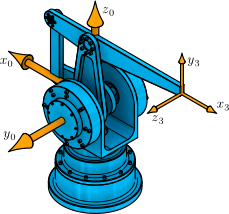This example computes the forward kinematics for the MIT Direct Drive Manipulator, which has a four-bar sub-chain.

## Clear All Workspace Objects and Reset All Assumptions

clear all


## Joint Values

theta1 = 180; % deg (motor angle)
theta2 = 90; % deg (motor angle)
phi3 = 180; % deg (motor angle)
theta3 = phi3 - theta2 - 180; % deg
theta23 = theta2 + theta3; % deg


## Structural Parameters

l2 = 0.4; % m
l3 = 0.4; % m


##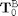: The Transformation from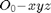to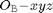T0B = [1 0 0 0.00;
0 1 0 0.00;
0 0 1 0.56;
0 0 0 1];


## Local Transformation Variables

U214 = l2*cosd(theta2) + l3*cosd(theta23);
U224 = l2*sind(theta2) + l3*sind(theta23);


##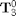: The Transformation from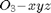toT30 = [cosd(theta1)*cosd(theta23) -cosd(theta1)*sind(theta23)  sind(theta1) U214*cosd(theta1);
sind(theta1)*cosd(theta23) -sind(theta1)*sind(theta23) -cosd(theta1) U214*sind(theta1);
sind(theta23)               cos(theta23)                0            U224;
0                           0                           0            1];


## The Forward Kinematics as the Homogeneous Transformation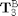T3B = T0B*T30

T3B =

-1.0000         0         0   -0.4000
0         0    1.0000         0
0    1.0000         0    0.9600
0         0         0    1.0000



This MATLAB example illustrates a computation from the textbook Fundamentals of Robot Mechanics by G. L. Long, Quintus-Hyperion Press, 2015. See http://www.RobotMechanicsControl.info for additional relevant files.